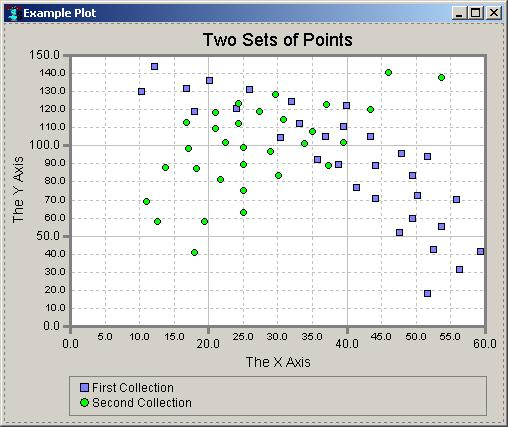## New plot-widget allows X/Y plots

The plot-widget is a facility for creating two-dimensional plots of X/Y coordinate pairs, such as scatter plots.

The plot-widget can be used to present static information or to dynamically monitor information as it changes. The widget will automatically lay out its axes and other parts (according to style properties that you can modify), and can also autocompute a rounded value range that encloses all of the data values.

The plot-widget is a sister widget of the chart-widget. Many of the classes are used by both and the plot-widget update modifed the class structure somewhat, and modified some existing methods by defining them on superclasses of the classes on which they were previously defined. Existing chart-widget code should work without change. The updated documentation reflects the new relationships.

### plot-widget example

We start with two 3-dimensional arrays of X/Y points. This represents two data sets which will be displayed (with different icons used for display) on a single plot:

```(defparameter *points*

;; Points for the first chart object.
#3a(((51.68 18.49) (59.28 41.71) (10.26 130.29) (12.16 144.05)
(20.14 136.31) (52.44 42.57) (49.4 59.77) (50.16 72.67)
(41.42 76.97) (35.72 92.45) (33.06 112.23) (39.52 110.51)
(47.88 95.89) (38.76 89.87) (25.84 131.15) (17.86 119.11)
(30.4 104.49) (44.08 89.01) (55.86 70.09) (47.5 52.03)
(44.08 70.95) (51.68 94.17) (36.86 105.35) (39.9 122.55)
(23.94 120.83) (16.72 132.01) (31.92 124.27) (56.24 31.39)
(53.58 55.47) (49.4 83.85) (43.32 105.35))

;; Points for the second chart object.
((37.0 123.0) (53.58 138.03) (45.98 140.61) (43.32 119.97)
(25.08 75.25) (24.32 123.41) (37.24 89.01) (39.52 101.91)
(34.96 107.93) (30.78 114.81) (24.32 112.23) (25.08 99.33)
(25.08 89.87) (20.9 109.65) (18.24 87.29) (11.02 69.23)
(12.54 58.05) (17.86 40.85) (19.38 58.05) (25.08 63.21)
(21.66 81.27) (13.68 88.15) (29.64 128.57) (20.9 118.25)
(16.72 113.09) (17.1 98.47) (22.42 101.91) (30.02 83.85)
(33.82 101.05) (28.88 96.75) (27.36 119.11))))
```

There are two ways to get a plot value for each point, the first calling set-plot-value and the second uses plot-value-returner. The example here uses the first method only. See the plot-widget for an example using the second method.

Now we create the plot-widget and a dialog to hold it. In a let* form, we create a plot-widget as the value of the variable plot-widget and then the dialog whose dialog-item is the plot-widget.

```(let* ((width 500)
(height 400)
(plot-widget
(make-instance 'plot-widget
:title "Two Sets of Points"
:chart-objects '((:id first :label "First Collection")
(:id second :label "Second Collection"))
:plot-view (make-instance 'plot-view
:icon-images '(:square :circle)
:draw-lines nil)
:x-axis (make-instance 'plot-value-axis
:axis-label "The X Axis")
:y-axis (make-instance 'plot-value-axis
:axis-label "The Y Axis")
:chart-legend (make-instance 'chart-legend
:layout-orientation :one-column)
:right-attachment :right
:bottom-attachment :bottom
:left 0 :top 0 :width width :height height))
(dialog (make-window :example-plot
:class 'dialog
:title "Example Plot"
:scrollbars nil
:interior (make-box-relative 40 40 width height)
:dialog-items (list plot-widget)))
item-id)

;; This version copies your data to the plot-widget,
;; one element at a time.
(dotimes (object-index (array-dimension *points* 0))
(dotimes (value-index (array-dimension *points* 1))
(set-plot-value plot-widget
:object-index object-index
:value-index value-index
:x (aref *points* object-index value-index 0)
:y (aref *points* object-index value-index 1))))
dialog)
```

We are displaying two data sets. The plot-view initarg to make-instance creates a plot-view instance with two icon-images (a square and a circle) to be used with the two data sets. The chart-objects initarg similarly has two elements, one for each data set.

Here is the plot displayed:The update which included the plot-widget functionality was released in the Fall of 2008, but the documentation has only recently been added.